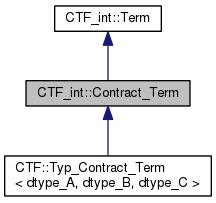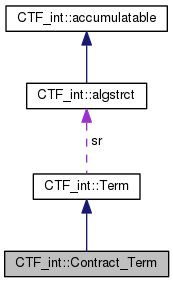Cyclops Tensor Framework parallel arithmetic on multidimensional arrays
CTF_int::Contract_Term Class Reference

An experession representing a contraction of a set of tensors contained in operands. More...

`#include <term.h>`

Inheritance diagram for CTF_int::Contract_Term:[legend]
Collaboration diagram for CTF_int::Contract_Term:[legend]

## Public Member Functions

Contract_Term (Term *B, Term *A)
creates sum term for B+A More...

~Contract_Term ()

Contract_Term (Contract_Term const &other, std::map< tensor *, tensor * > *remap=NULL)

Termclone (std::map< tensor *, tensor * > *remap=NULL) const
base classes must implement this copy function to retrieve pointer More...

void execute (CTF::Idx_Tensor output) const
override execution to to contract operands and add them to output More...

std::vector< char > get_uniq_inds () const
find list of unique indices that are involved in this term More...

void get_inputs (std::set< CTF::Idx_Tensor *, tensor_name_less > *inputs_set) const
appends the tensors this depends on to the input set More...

CTF::Idx_Tensor execute (std::vector< char > out_inds) const
evalues the expression to produce an intermediate with all expression indices remaining More...

double estimate_time (CTF::Idx_Tensor output) const
estimates the cost of a contract term More...

CTF::Idx_Tensor estimate_time (double &cost, std::vector< char > out_inds) const
estimates the cost the expression to produce an intermediate with all expression indices remaining More...

Contract_Term operator* (Term const &A) const
override contraction to grow vector rather than create recursive terms More...

CTF::Worldwhere_am_i () const
negates term More...Public Member Functions inherited from CTF_int::Term
Term (algstrct const *sr)

virtual ~Term ()

void mult_scl (char const *mulscl)
multiply scaling factor by mulscl More...

Contract_Term operator* (Term const &A) const
constructs a new term which multiplies by tensor A More...

Contract_Term operator* (int64_t scl) const
multiples by a constant More...

Contract_Term operator* (double scl) const

Sum_Term operator+ (Term const &A) const
constructs a new term by addition of two terms More...

Sum_Term operator+ (double scl) const

Sum_Term operator+ (int64_t scl) const

Sum_Term operator- (Term const &A) const
constructs a new term by subtracting term A More...

Sum_Term operator- (double scl) const

Sum_Term operator- (int64_t scl) const

Termoperator- ()

void operator= (CTF::Idx_Tensor const &B)
A = B, compute any operations on operand B and set. More...

void operator= (Term const &B)

void operator+= (Term const &B)

void operator-= (Term const &B)

void operator*= (Term const &B)

void operator= (double scl)

void operator+= (double scl)

void operator<< (CTF_int::Term const &B)

void operator<< (double scl)

void operator-= (double scl)

void operator*= (double scl)

void operator= (int64_t scl)

void operator+= (int64_t scl)

void operator-= (int64_t scl)

void operator*= (int64_t scl)

void operator= (int scl)

void operator+= (int scl)

void operator-= (int scl)

void operator*= (int scl)

operator float () const
cast to float (works only if tensor type is castable to float) allows a scalar output More...

operator double () const
cast to double (works only if tensor type is castable to double) allows a scalar output More...

operator int64_t () const
cast to int64_t (works only if tensor type is castable to int64_t) allows a scalar output More...

operator int () const
cast to int64_t (works only if tensor type is castable to int64_t) allows a scalar output More...

## Data Fields

std::vector< Term * > operandsData Fields inherited from CTF_int::Term
char * scale

algstrctsr

## Detailed Description

An experession representing a contraction of a set of tensors contained in operands.

Definition at line 275 of file term.h.

## Constructor & Destructor Documentation

 CTF_int::Contract_Term::Contract_Term ( Term * B, Term * A )

creates sum term for B+A

Parameters
 [in] B left hand side [in] A right hand side

Definition at line 535 of file term.cxx.

References operands.

Referenced by clone().

 CTF_int::Contract_Term::~Contract_Term ( )

Definition at line 516 of file term.cxx.

References CTF_int::Sum_Term::operands.

 CTF_int::Contract_Term::Contract_Term ( Contract_Term const & other, std::map< tensor *, tensor * > * remap = `NULL` )

Definition at line 541 of file term.cxx.

## Member Function Documentation

 Term * CTF_int::Contract_Term::clone ( std::map< tensor *, tensor * > * remap = `NULL` ) const
virtual

base classes must implement this copy function to retrieve pointer

Implements CTF_int::Term.

Definition at line 552 of file term.cxx.

References Contract_Term().

Referenced by CTF_int::contract_down_terms().

 double CTF_int::Contract_Term::estimate_time ( CTF::Idx_Tensor output ) const
virtual

estimates the cost of a contract term

Parameters
 [in] output tensor to write results into and its indices

Implements CTF_int::Term.

Definition at line 677 of file term.cxx.

 Idx_Tensor CTF_int::Contract_Term::estimate_time ( double & cost, std::vector< char > out_inds ) const
virtual

estimates the cost the expression to produce an intermediate with all expression indices remaining

Parameters
 [in,out] cost the cost of the operation [in] out_inds unique indices to not contract/sum away
Returns
output tensor to write results into and its indices

Implements CTF_int::Term.

Definition at line 718 of file term.cxx.

 void CTF_int::Contract_Term::execute ( CTF::Idx_Tensor output ) const
virtual

override execution to to contract operands and add them to output

Parameters
 [in,out] output tensor to write results into and its indices

Implements CTF_int::Term.

Definition at line 616 of file term.cxx.

Referenced by CTF_int::Term::operator<<().

 Idx_Tensor CTF_int::Contract_Term::execute ( std::vector< char > out_inds ) const
virtual

evalues the expression to produce an intermediate with all expression indices remaining

Parameters
 [in] out_inds unique indices to not contract/sum away
Returns
output tensor to write results into and its indices

Implements CTF_int::Term.

Definition at line 664 of file term.cxx.

 void CTF_int::Contract_Term::get_inputs ( std::set< CTF::Idx_Tensor *, tensor_name_less > * inputs_set ) const
virtual

appends the tensors this depends on to the input set

Implements CTF_int::Term.

Definition at line 727 of file term.cxx.

References operands.

 std::vector< char > CTF_int::Contract_Term::get_uniq_inds ( ) const
virtual

find list of unique indices that are involved in this term

Returns
out_inds unique indices to not contract/sum away

Implements CTF_int::Term.

Definition at line 723 of file term.cxx.

References CTF_int::det_uniq_inds(), and operands.

Referenced by CTF_int::Term::operator<<().

 Contract_Term CTF_int::Contract_Term::operator* ( Term const & A ) const

override contraction to grow vector rather than create recursive terms

Parameters
 [in] A term to multiply by

Definition at line 557 of file term.cxx.

References CTF_int::Term::clone(), and operands.

 World * CTF_int::Contract_Term::where_am_i ( ) const
virtual

negates term

figures out what world this term lives on

Implements CTF_int::Term.

Definition at line 524 of file term.cxx.

## Field Documentation

 std::vector< Term* > CTF_int::Contract_Term::operands

Definition at line 277 of file term.h.

Referenced by Contract_Term(), estimate_time(), execute(), get_inputs(), get_uniq_inds(), and operator*().

The documentation for this class was generated from the following files: